# Quick Piece Flying Geese

#### by Debby Kratovil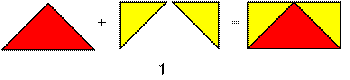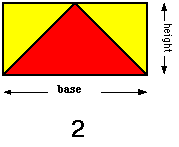The Flying Geese unit is composed of three triangles which together form a rectangle (see Figure #1). The goose is usually an equilateral triangle. Its height is 1/2 the measurement of its base/width. The skies are right triangles, with their measurement that of the goose height. When you know the finished measurements of this unit, you can piece it in any size (see Figure #2) Flying Geese are a breeze to piece with the following two simple formulas. Both the goose triangles and the sky triangles are cut from squares.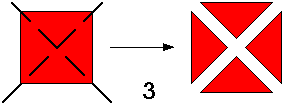For the goose triangle, cut a square of fabric that measures 1-1/4" larger than the size of the finished base. For example, for a finished width of 5 inches, I will cut a 5" plus 1-1/4" square of geese fabric. Cut this square twice diagonally (see Figure #3). This gives you 4 geese triangles.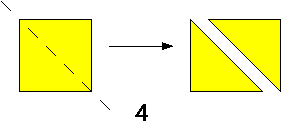For the sky triangles, cut a square 7/8" larger than the size of the height of the unit (which is half the base/width). Cut this square once on the diagonal (see Figure #4). You have two sky triangles, enough for one unit.

What are those formulas again? The goose is cut from a square that is 1-1/4" larger than the finished base of the Flying Geese unit (which is cut into 4 equal sized triangles). The sky is cut from a square that is 7/8" larger than the finished height of the Flying Geese unit (which is cut into 2 equal sized triangles). See the chart below for the formulas for some standard sized Flying Geese units. And happy flying...I mean quilting!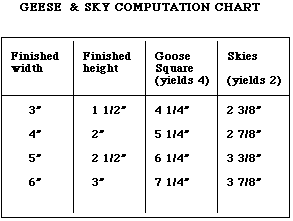Back to Quilting Lessons page